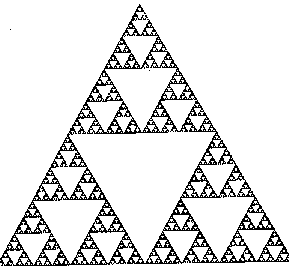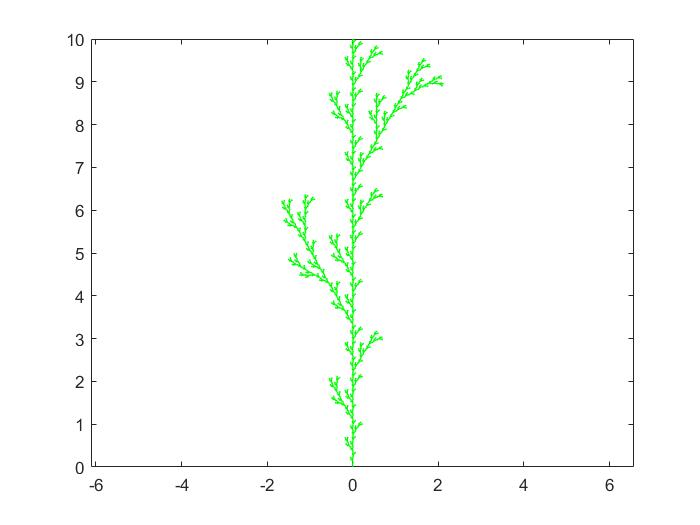# 迭代与分形# 实验过程

## Koch曲线## Matlab代码

function plotkoch(k)      %显示迭代k次后的Koch曲线图
p=[0,0;10,0]; %存放结点坐标，每行一个点，初始值为两结点的坐标
n=1;    %存放线段的数量，初始值为1（思考：n若为结点数，后续该如何处理
A=[cos(pi/3),-sin(pi/3);sin(pi/3),cos(pi/3)]; %用于计算新的结点%旋转
for s=1:k     %实现迭代过程，计算所有的结点的坐标
j=0;       % 思考：可否取为1

%以下根据线段两个结点的坐标，计算迭代后它们之间增加的三个
%结点的坐标，并且将这些点的坐标按次序存暂时放到r中
for i=1:n              %每条边计算一次
q1=p(i,:);          %目前线段的起点坐标
q2=p(i+1,:);      %目前线段的终点坐标
d=(q2-q1)/3;
j=j+1;r(j,:)=q1;                %原起点存入r
j=j+1;r(j,:)=q1+d;            %新1点存入r
j=j+1;r(j,:)=q1+d+d*A';   %新2点存入r
j=j+1;r(j,:)=q1+2*d;        %新3点存入r
end  %原终点作为下条线段的起点，在迭代下条线段时存入r

n=4*n;    %全部线段迭代一次后，线段数量乘4
clear p    %清空p ，注意：最后一个终点q2不在r中
p=[r;q2];   %重新装载本次迭代后的全部结点
end

figure
plot(p(:,1),p(:,2))   %显示各结点的连线图
axis equal              %各坐标轴同比例（思考：若没有这项操作会怎样？）


function koch(k)
p=[0 10]; %存放结点坐标
for m=1:k  %实现迭代过程，计算所有的结点的坐标
q1=p/3;  %将上一次迭代结果缩小到1/3
q2=10/3+(q1*exp(i*pi/3));  %斜向右上部分
q3=(10/3+10/3*exp(1i*pi/3))+(q1*exp(-1i*pi/3)); %斜向右下部分
q4=20/3+p/3; %右边水平部分
p=[q1 q2 q3 q4];
end
figure
plot(p)               %显示各结点的连线图
axis equal          %各坐标轴同比例


# 课后练习

## Koch雪花

function koch(k)
p=[0 10]; %存放结点坐标
for m=1:k  %实现迭代过程，计算所有的结点的坐标
q1=p/3;  %将上一次迭代结果缩小到1/3
q2=10/3+(q1*exp(i*pi/3));  %斜向右上部分
q3=(10/3+10/3*exp(1i*pi/3))+(q1*exp(-1i*pi/3)); %斜向右下部分
q4=20/3+p/3; %右边水平部分
p=[q1 q2 q3 q4];
end
p1=p*exp(2*i*pi/3)+5-5*sqrt(3)*i; %逆时针旋转120度后平移
p2=p;
p3=p*exp(-2*i*pi/3)+10; %顺时针旋转120度后平移
p_all=[p1 p2 p3];
figure
plot(p_all)               %显示各结点的连线图
axis equal          %各坐标轴同比例## 树

function koch(k)
p=[0 1i*10]; %存放结点坐标
for m=1:k  %实现迭代过程，计算所有的结点的坐标
q1=p/3;  %将上一次迭代结果缩小到1/3
q2=1i*10/3+(q1*exp(1i*pi/6));  %斜向左上部分
q3=1i*10/3+q1; %斜向右下部分
q4=1i*20/3+(q1*exp(-1i*pi/6)); %斜向右上部分
q5=1i*20/3+q1;
p=[q1 q2 q3 q4 q5];
end
figure
plot(p, 'g')               %显示各结点的连线图
axis equal          %各坐标轴同比例
hold off